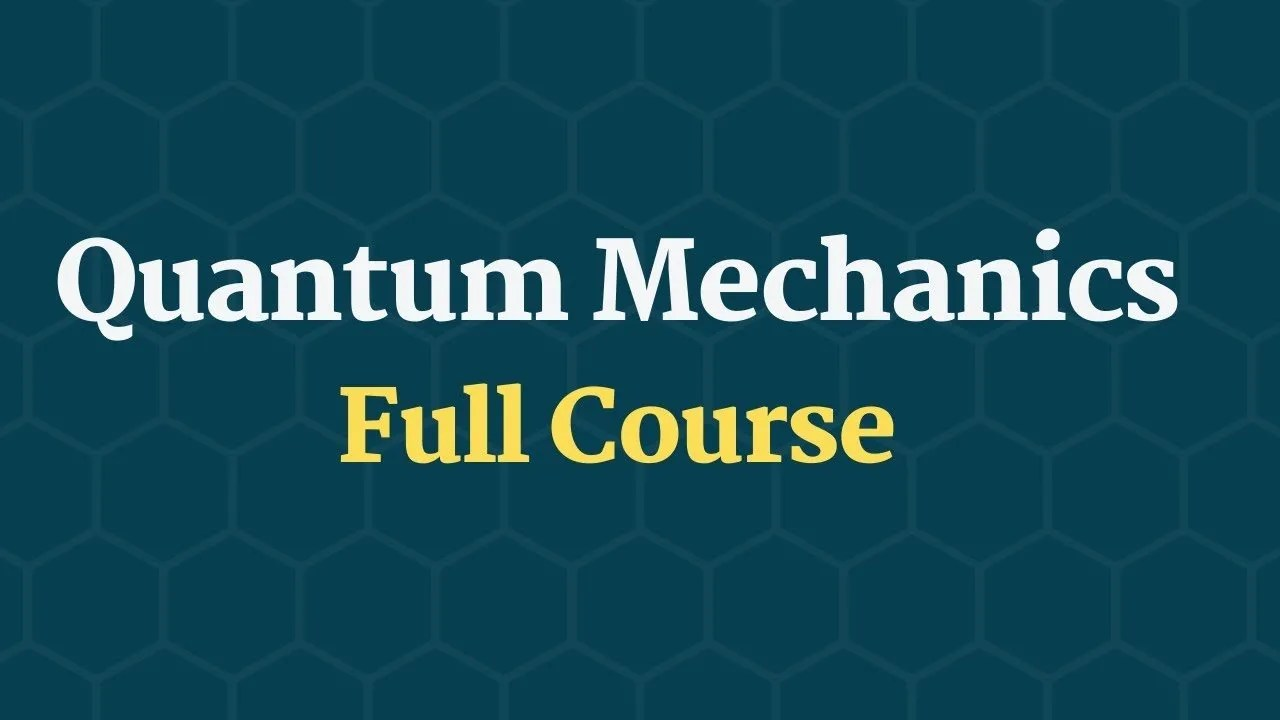# Quantum Physics Full CourseHere’ is a great (and free) course covering quantum mechanics.

Quantum physics also known as Quantum mechanics is a fundamental theory in physics that provides a description of the physical properties of nature at the scale of atoms and subatomic particles. It is the foundation of all #quantum #physics including quantum chemistry, quantum field theory, quantum technology, and quantum information science.

• (0:00:00) Introduction to quantum mechanics
• (0:16:23) The domain of quantum mechanics
• (0:24:18) Key concepts of quantum mechanics
• (0:34:04) A review of complex numbers for QM
• (0:48:12) Examples of complex numbers
• (1:01:47) Probability in quantum mechanics
• (1:12:17) Variance of probability distribution
• (1:26:16) Normalization of wave function
• (1:51:47) Position, velocity and momentum from the wave function
• (2:10:59) Introduction to the uncertainty principle
• (2:24:32) Key concepts of QM – revisited
• (2:37:45) Separation of variables and Schrodinger equation
• (3:09:55) Stationary solutions to the Schrodinger equation
• (3:15:47) Superposition of stationary states
• (3:25:37) Potential function in the Schrodinger equation
• (3:48:10) Infinite square well (particle in a box)
• (4:00:58) Infinite square well states, orthogonality – Fourier series
• (4:08:07) Infinite square well example – computation and simulation
• (4:39:27) Quantum harmonic oscillators via ladder operators
• (5:16:48) Quantum harmonic oscillators via power series
• (5:28:32) Free particles and Schrodinger equation
• (5:34:37) Free particles wave packets and stationary states
• (6:10:33) Free particle wave packet example
• (6:13:43) The Dirac delta function
• (6:20:49) Boundary conditions in the time independent Schrodinger equation
• (6:24:39) The bound state solution to the delta function potential TISE
• (6:43:29) Scattering delta function potential
• (6:55:49) Finite square well scattering states
• (7:07:39) Linear algebra introduction for quantum mechanics
• (7:10:34) Linear transformation
• (7:13:04) Mathematical formalism is Quantum mechanics
• (7:37:52) Hermitian operator eigen-stuff
• (8:01:23) Statistics in formalized quantum mechanics
• (8:24:26) Generalized uncertainty principle
• (8:54:36) Energy time uncertainty
• (9:16:33) Schrodinger equation in 3d
• (9:19:56) Hydrogen spectrum
• (9:31:14) Angular momentum operator algebra
• (9:57:17) Angular momentum eigen function
• (10:18:08) Spin in quantum mechanics
• (10:22:23) Two particles system
• (10:58:03) Free electrons in conductors
• (11:09:23) Band structure of energy levels in solids